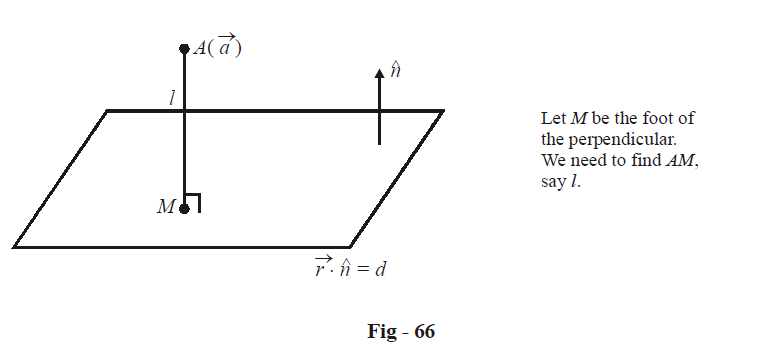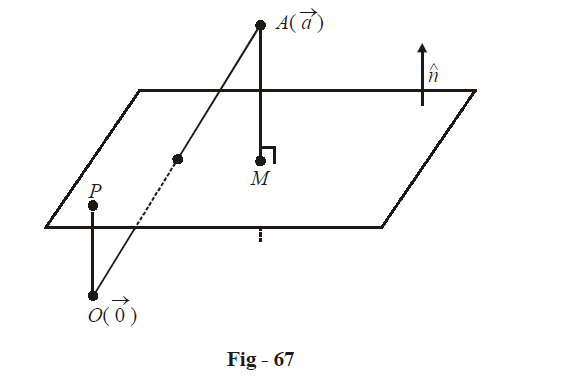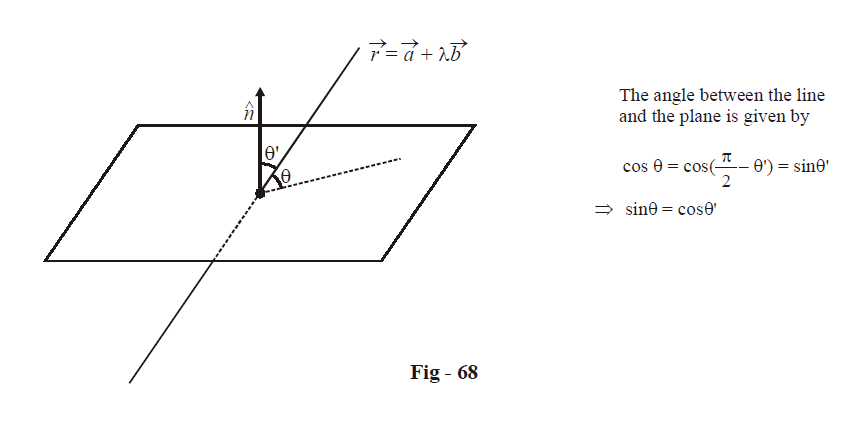# Examples On Vector Equations Of Planes Set-1

Go back to  'Vectors and 3-D Geometry'

Example – 51

Find the length of the perpendicular dropped from the point $$A(\vec a)$$ onto the plane $$\vec r \cdot \hat n = d.$$

Solution:Let O be the origin. The position vector of M can be written as

\begin{align}& {{\vec r}_M} = \overrightarrow {OA} + \overrightarrow {AM} \hfill \\\\&\quad\; = \vec a - l\,\hat n\;\;\;\qquad where\;\;l = AM \hfill \\ \end{align}

Since M lies on the plane, we must have

\begin{align}&\qquad\;\; {{\vec r}_M} \cdot \hat n = d \hfill \\\\&\Rightarrow \quad (\vec a - l\,\hat n) \cdot \hat n = d \hfill \\\\&\Rightarrow \quad \vec a \cdot \hat n - l = d \hfill \\\\& \Rightarrow \quad l = \vec a \cdot \hat n - d \hfill \\ \end{align}

Thus, the length AM is  $$\left| {\vec a \cdot \hat n - d} \right|.$$

We could have evaluated this result straightaway by inspection using a slightly more elaborate diagram.

In particular, let us include the origin  $$O(\vec 0)$$ in our diagram:OP, the perpendicular from O onto the plane, is of length d since the equation of the plane is $$\vec r \cdot \hat n = d.$$

The component of OA along the extended line AM, say $$O{A_{AM}},$$ will be $$\vec a \cdot \hat n.$$ Thus,

\begin{align}&AM = \left| {O{A_{AM}} - OP} \right| \hfill \\\\&\qquad= \left| {\vec a \cdot \hat n - d} \right| \hfill \\ \end{align}

Example – 52

(a) Find the angle between the line $$\vec r = \vec a + \lambda \vec b$$ and the plane $$\vec r \cdot \hat n = d.$$

(b) Find the angle between the planes $$\vec r \cdot {\hat n_1} = {d_1}$$ and $$\vec r \cdot {\hat n_2} = {d_2}.$$

Solution:(a)$${{\theta '}}$$  is simply the angle between the vectors  $$\hat n$$ and $$\vec b.$$

Thus,

$\cos {{\theta '}} = \frac{{\vec b \cdot \hat n}}{{\left| {\vec b} \right|\,\,\left| {\hat n} \right|}} = \hat b \cdot \hat n$

The angle between the line and the plane is therefore given by

\begin{align}&\quad\quad\sin {{\theta }} = \cos {{\theta '}} = \hat b \cdot \hat n \hfill \\\\&\;\;\; \Rightarrow \quad {{\theta }} = {\sin ^{ - 1}}\left( {\hat b \cdot \hat n} \right) \hfill \\ \end{align}

(b) The angle $${\theta }$$ between the two planes will simply be the angle between their normals. Thus,

\begin{align}&\qquad\quad\cos {{\theta }} = \frac{{{{\hat n}_1} \cdot {{\hat n}_2}}}{{\left| {{{\hat n}_1}} \right|\left| {{{\hat n}_2}} \right|}} = {{\hat n}_1}.{{\hat n}_2} \hfill \\& \Rightarrow \quad {{\theta }} = {\cos ^{ - 1}}\left( {{{\hat n}_1} \cdot {{\hat n}_2}} \right) \hfill \\ \end{align}

Vectors
grade 11 | Questions Set 1
Vectors